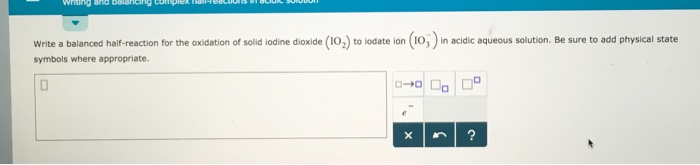Chemistry Practice Problems Balancing Redox Reactions Practice Problems Solution: Write a balanced half-reaction for the oxidation o...

🤓 Based on our data, we think this question is relevant for Professor Joseph's class at UCSB.

# Solution: Write a balanced half-reaction for the oxidation of solid iodine dioxide (IO2) to iodate ion (IO3-) in acidic aqueous solution. Be sure to odd physical state symbols where appropriate.

###### Problem

Write a balanced half-reaction for the oxidation of solid iodine dioxide (IO2) to iodate ion (IO3-) in acidic aqueous solution. Be sure to odd physical state symbols where appropriate.###### Solution

First step in balancing redox half reactions is that try to balance first the non- Hs and Os in the reaction

For IO2 and IO3-, I is already balanced. Next step is balance the O by adding H2O in either of the sides

In this case, we have to add H2O in the reactant sideView Complete Written Solution

Balancing Redox Reactions

Balancing Redox Reactions

#### Q. Balance each of the following equations according to the half-reaction method:(b) H2S(g) + Hg22+(aq) ⟶ Hg(l) + S(s) (in acid)

Solved • Thu Oct 18 2018 12:41:28 GMT-0400 (EDT)

Balancing Redox Reactions

#### Q. Complete and balance each of the following half-reactions (steps 2–5 in half-reaction method):(b) Hg(l) + Br−(aq) ⟶ HgBr42−(aq)

Solved • Thu Oct 18 2018 12:23:21 GMT-0400 (EDT)

Balancing Redox Reactions

#### Q. (1) Identify each of the following half-reactions as either an  oxidation half-reaction or a reduction half-reaction. (2) Write a balanced equation fo...

Solved • Tue Aug 28 2018 11:54:54 GMT-0400 (EDT)

Balancing Redox Reactions

#### Q. (1) Identify each of the following half-reactions as either an  oxidation half-reaction or a reduction half-reaction. (2) Write a balanced equation fo...

Solved • Tue Aug 28 2018 11:49:08 GMT-0400 (EDT)